# Long-Term Uplift Rates

This page displays long-term rates of uplift or subsidence, calculated using data from the Bottom Pressure Recorders (NANO-BPR) on the BOTPT instruments at Axial Seamount, part of the National Science Foundation's (NSF) Ocean Observatories Initiative (OOI) Cabled Array seafloor observatory, operated by the University of Washington. The dates/times below are in GMT (+8 hrs of local standard time, or +7 hrs of DLS time on the US west coast). Note the long-term rates were reset after the April 2015 eruption.

The 12-week uplift rate from the Central Caldera (MJ03F, 1st plot below) is used on the Forecasting - Method #1 page. However, we have another forecasting method that is probably better on the Forecasting - Method #2 page, which used the Rate of Differential Uplift between stations MJ03F and MJ03E. This removes the "noise" from tidal residuals and oceanographic effects and provides a clearer representation of the volcanic inflation signal.

## BOTPT-A301-MJ03F - Central Caldera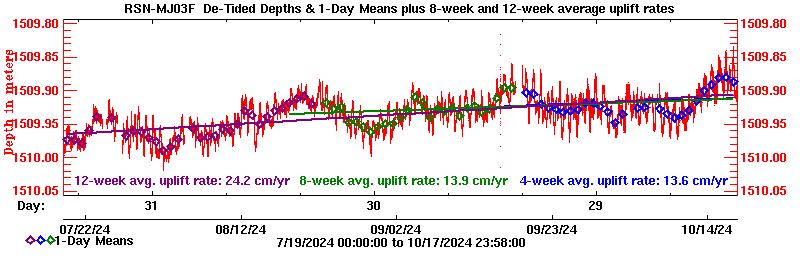Plot 1: Plot of long-term rates of depth change calculated from the last 4-weeks, 8-weeks, and 12-weeks.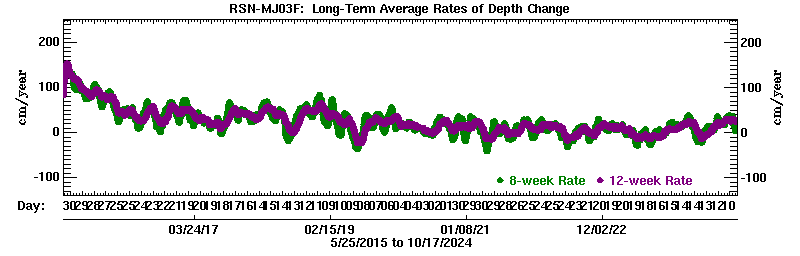Plot 2: Plot of how the long-term rates have varied over time, since 25 May 2015.

## BOTPT-A302-MJ03E - Eastern Caldera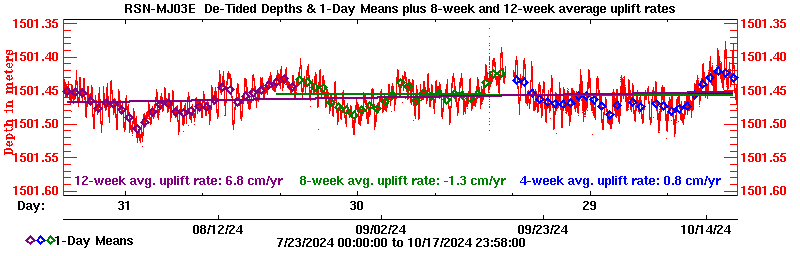Plot 3: Plot of long-term rates of depth change calculated from the last 4-weeks, 8-weeks, and 12-weeks.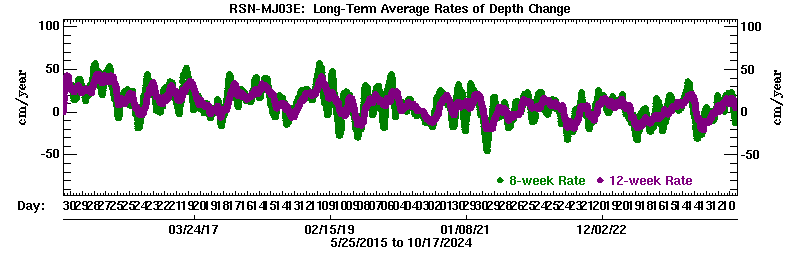Plot 4: Plot of how the long-term rates have varied over time, since 25 May 2015.

## BOTPT-A303-MJ03D - International DistrictPlot 5: Plot of long-term rates of depth change calculated from the last 4-weeks, 8-weeks, and 12-weeks.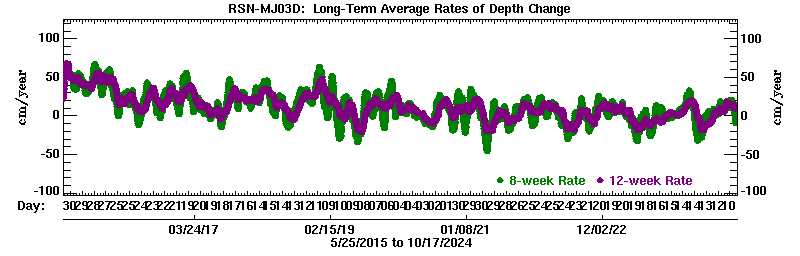Plot 6: Plot of how the long-term rates have varied over time, since 25 May 2015.

## BOTPT-A304-MJ03B - ASHES Vent field (deployed mid-August 2017)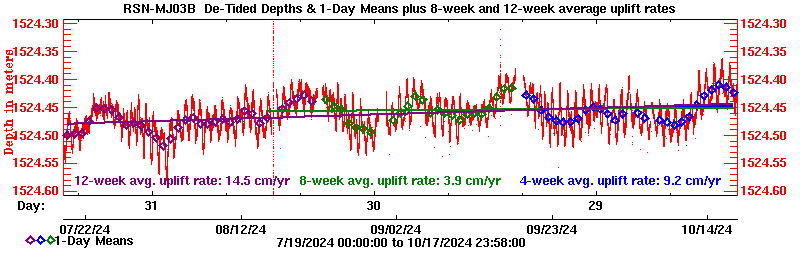Plot 7: Plot of long-term rates of depth change calculated from the last 4-weeks, 8-weeks, and 12-weeks.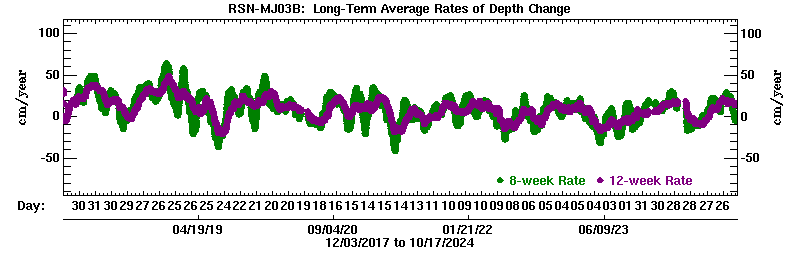Plot 8: Plot of how the long-term rates have varied over time, since 03 Dec 2017.

## BOTPT-A301-MJ03F - Central Caldera - 25 April to 05 May 2015 ONLY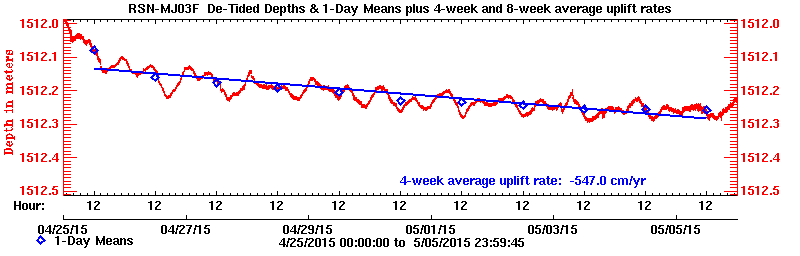Plot 9: Plot of long-term rates of depth change calculated from 25 April to 05 May 2015.

## BOTPT-A302-MJ03E - Eastern Caldera - 25 April to 05 May 2015 ONLY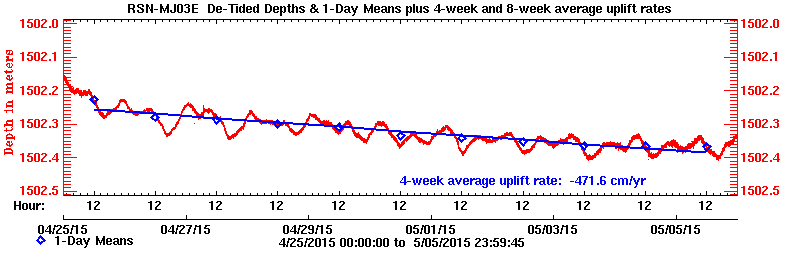Plot 10: Plot of long-term rates of depth change calculated from 25 April to 05 May 2015.

## BOTPT-A303-MJ03D - International District - 25 April to 05 May 2015 ONLY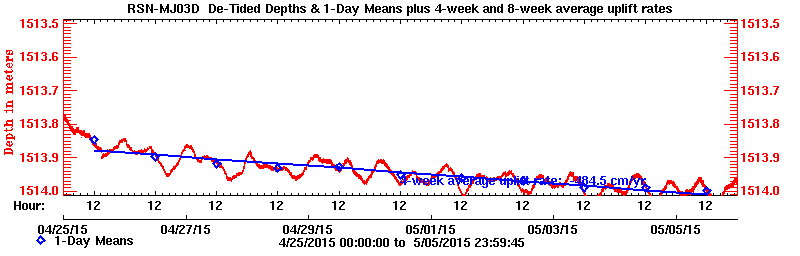Plot 11: Plot of long-term rates of depth change calculated from 25 April to 05 May 2015.

## BOTPT-A301-MJ03F - Central Caldera - BEFORE 24 April 2015 ONLY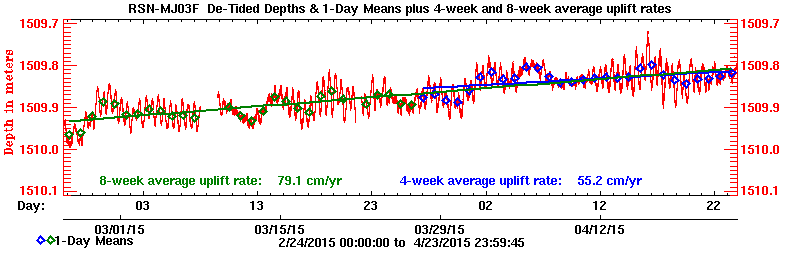Plot 12: Plot of long-term rates of depth change calculated from the last 4- and 8-weeks, before 24 April 2015.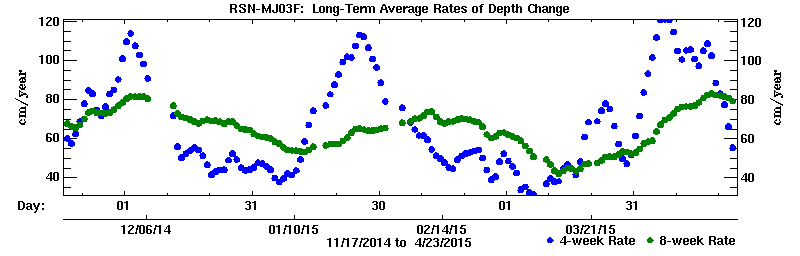Plot 13: Plot of how the long-term rates have varied over time, before 24 April 2015.

## BOTPT-A302-MJ03E - Eastern Caldera - BEFORE 24 April 2015 ONLY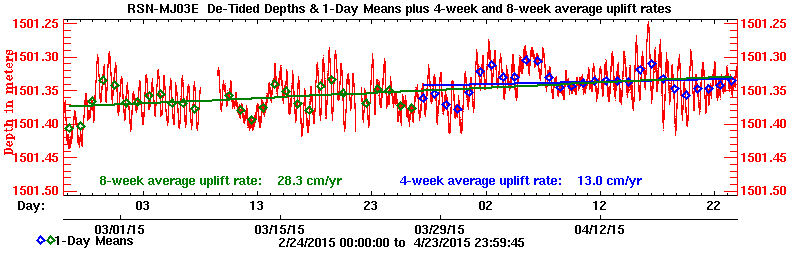Plot 14: Plot of long-term rates of depth change calculated from the last 4- and 8-weeks, before 24 April 2015.Plot 15: Plot of how the long-term rates have varied over time, before 24 April 2015.

## BOTPT-A303-MJ03D - International District - BEFORE 24 April 2015 ONLY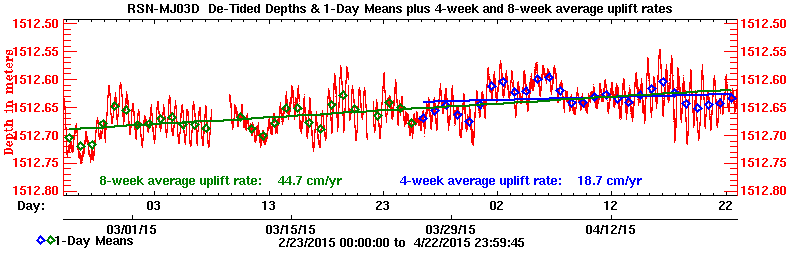Plot 16: Plot of long-term rates of depth change calculated from the last 4- and 8-weeks, before 24 April 2015.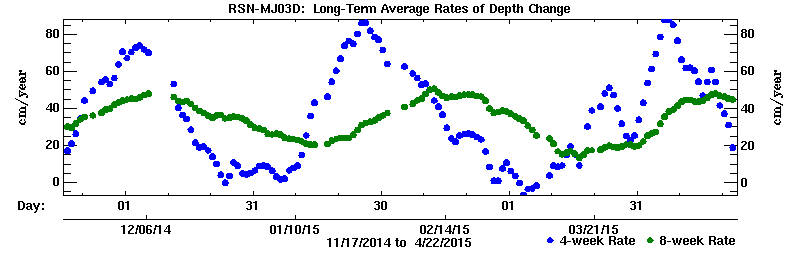Plot 17: Plot of how the long-term rates have varied over time, before 24 April 2015.